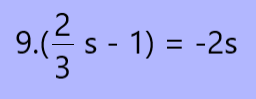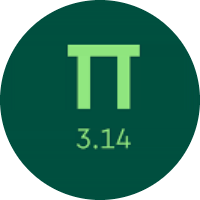# Practicing the One Variable Equations

In this quiz, you will get to know about the one variable equations. You will find questions on writing equations, finding the variables, checking the variable whether it satisfies the equation or not, and solving the one-step, and two-step equations.

Start Quiz

What value of v is a solution to this equation?

40 = -6(v - 9) - 8

v = 1

v = 2

Which property of equality is shown below?

If: 92 = 32 − c

Then: 92 − d = 32 − c − d

Subtraction Property of Equality

Multiplication Property of Equality

Division Property of Equality

Select all of the equations below that are equivalent to:
5 = f + g
Use properties of equality.

17 = 14 + f + g

15 = f + g + 10

20 = f + g + 13

17 = 12 + f + g

Solve for b.

b + 48 = 73

b = 25

b = 15

b = 35

b = 23

Solve for p.

12p + 7p - 3p = 16

p = 1

p = -1

p = 2

p = -2

Solve for s.

8 + 7s + 7 = - 9s - 1

s = 2

s = -1

s = -2

Solve for r.

21r = 7(2r + 9)

r = 3

r = 4

r = 9

r = 5

Solve for x.

-7(-5x + 10) + 1 = 10 + 6x + 8

x = 5

x = 3

x = 4

x = 2

Solve for s.s = 3/4

s = 2/3

s = 9/8

s = 3/4

How many solutions does this equation have?

–4 − 6r = –6r

no solution

one solution

infinitely many solutions

Quiz/Test Summary
Title: Practicing the One Variable Equations
Questions: 10
Contributed by: